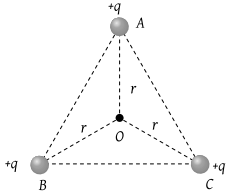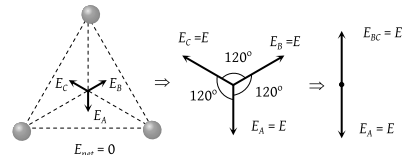NEET Questions Solved

PMT - 1985

ABC is an equilateral triangle. Charges +q are placed at each corner. The electric intensity at O will be(1) $\frac{1}{4\pi {\epsilon }_{0}}\frac{q}{{r}^{2}}$

(2) $\frac{1}{4\pi {\epsilon }_{0}}\frac{q}{r}$

(3) Zero

(4) $\frac{1}{4\pi {\epsilon }_{0}}\frac{3q}{{r}^{2}}$

(3)Difficulty Level:

• 4%
• 5%
• 81%
• 12%